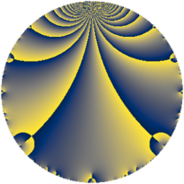# Properties

 Label 2672.2.a.cLevel $2672$ Weight $2$ Character orbit 2672.a Self dual yes Analytic conductor $21.336$ Analytic rank $1$ Dimension $2$ CM no Inner twists $1$

# Related objects

## Newspace parameters

 Level: $$N$$ $$=$$ $$2672 = 2^{4} \cdot 167$$ Weight: $$k$$ $$=$$ $$2$$ Character orbit: $$[\chi]$$ $$=$$ 2672.a (trivial)

## Newform invariants

 Self dual: yes Analytic conductor: $$21.3360274201$$ Analytic rank: $$1$$ Dimension: $$2$$ Coefficient field: $$\Q(\sqrt{13})$$ Defining polynomial: $$x^{2} - x - 3$$ Coefficient ring: $$\Z[a_1, a_2, a_3]$$ Coefficient ring index: $$1$$ Twist minimal: no (minimal twist has level 668) Fricke sign: $$1$$ Sato-Tate group: $\mathrm{SU}(2)$

## $q$-expansion

Coefficients of the $$q$$-expansion are expressed in terms of $$\beta = \frac{1}{2}(1 + \sqrt{13})$$. We also show the integral $$q$$-expansion of the trace form.

 $$f(q)$$ $$=$$ $$q -\beta q^{3} -3 q^{5} + ( -1 - \beta ) q^{7} + \beta q^{9} +O(q^{10})$$ $$q -\beta q^{3} -3 q^{5} + ( -1 - \beta ) q^{7} + \beta q^{9} + ( 4 + \beta ) q^{13} + 3 \beta q^{15} + ( 1 - \beta ) q^{17} -2 q^{19} + ( 3 + 2 \beta ) q^{21} + ( 1 - \beta ) q^{23} + 4 q^{25} + ( -3 + 2 \beta ) q^{27} + ( 5 - 2 \beta ) q^{29} + ( -4 + 2 \beta ) q^{31} + ( 3 + 3 \beta ) q^{35} + ( 3 + 2 \beta ) q^{37} + ( -3 - 5 \beta ) q^{39} + ( -1 + 4 \beta ) q^{41} + ( -7 + 2 \beta ) q^{43} -3 \beta q^{45} + ( 1 + 2 \beta ) q^{47} + ( -3 + 3 \beta ) q^{49} + 3 q^{51} + ( -2 + 2 \beta ) q^{53} + 2 \beta q^{57} + 6 \beta q^{59} + ( -1 - 6 \beta ) q^{61} + ( -3 - 2 \beta ) q^{63} + ( -12 - 3 \beta ) q^{65} + ( -3 + 4 \beta ) q^{67} + 3 q^{69} + 3 \beta q^{71} + ( -7 - 3 \beta ) q^{73} -4 \beta q^{75} + ( -9 - 2 \beta ) q^{79} + ( -6 - 2 \beta ) q^{81} + ( -1 - 2 \beta ) q^{83} + ( -3 + 3 \beta ) q^{85} + ( 6 - 3 \beta ) q^{87} + ( -6 + 6 \beta ) q^{89} + ( -7 - 6 \beta ) q^{91} + ( -6 + 2 \beta ) q^{93} + 6 q^{95} + ( -2 - 5 \beta ) q^{97} +O(q^{100})$$ $$\operatorname{Tr}(f)(q)$$ $$=$$ $$2q - q^{3} - 6q^{5} - 3q^{7} + q^{9} + O(q^{10})$$ $$2q - q^{3} - 6q^{5} - 3q^{7} + q^{9} + 9q^{13} + 3q^{15} + q^{17} - 4q^{19} + 8q^{21} + q^{23} + 8q^{25} - 4q^{27} + 8q^{29} - 6q^{31} + 9q^{35} + 8q^{37} - 11q^{39} + 2q^{41} - 12q^{43} - 3q^{45} + 4q^{47} - 3q^{49} + 6q^{51} - 2q^{53} + 2q^{57} + 6q^{59} - 8q^{61} - 8q^{63} - 27q^{65} - 2q^{67} + 6q^{69} + 3q^{71} - 17q^{73} - 4q^{75} - 20q^{79} - 14q^{81} - 4q^{83} - 3q^{85} + 9q^{87} - 6q^{89} - 20q^{91} - 10q^{93} + 12q^{95} - 9q^{97} + O(q^{100})$$

## Embeddings

For each embedding $$\iota_m$$ of the coefficient field, the values $$\iota_m(a_n)$$ are shown below.

For more information on an embedded modular form you can click on its label.

Label $$\iota_m(\nu)$$ $$a_{2}$$ $$a_{3}$$ $$a_{4}$$ $$a_{5}$$ $$a_{6}$$ $$a_{7}$$ $$a_{8}$$ $$a_{9}$$ $$a_{10}$$
1.1
 2.30278 −1.30278
0 −2.30278 0 −3.00000 0 −3.30278 0 2.30278 0
1.2 0 1.30278 0 −3.00000 0 0.302776 0 −1.30278 0
 $$n$$: e.g. 2-40 or 990-1000 Significant digits: Format: Complex embeddings Normalized embeddings Satake parameters Satake angles

## Atkin-Lehner signs

$$p$$ Sign
$$2$$ $$-1$$
$$167$$ $$-1$$

## Inner twists

This newform does not admit any (nontrivial) inner twists.

## Twists

By twisting character orbit
Char Parity Ord Mult Type Twist Min Dim
1.a even 1 1 trivial 2672.2.a.c 2
4.b odd 2 1 668.2.a.a 2
12.b even 2 1 6012.2.a.a 2

By twisted newform orbit
Twist Min Dim Char Parity Ord Mult Type
668.2.a.a 2 4.b odd 2 1
2672.2.a.c 2 1.a even 1 1 trivial
6012.2.a.a 2 12.b even 2 1

## Hecke kernels

This newform subspace can be constructed as the intersection of the kernels of the following linear operators acting on $$S_{2}^{\mathrm{new}}(\Gamma_0(2672))$$:

 $$T_{3}^{2} + T_{3} - 3$$ $$T_{7}^{2} + 3 T_{7} - 1$$

## Hecke characteristic polynomials

$p$ $F_p(T)$
$2$ $$T^{2}$$
$3$ $$-3 + T + T^{2}$$
$5$ $$( 3 + T )^{2}$$
$7$ $$-1 + 3 T + T^{2}$$
$11$ $$T^{2}$$
$13$ $$17 - 9 T + T^{2}$$
$17$ $$-3 - T + T^{2}$$
$19$ $$( 2 + T )^{2}$$
$23$ $$-3 - T + T^{2}$$
$29$ $$3 - 8 T + T^{2}$$
$31$ $$-4 + 6 T + T^{2}$$
$37$ $$3 - 8 T + T^{2}$$
$41$ $$-51 - 2 T + T^{2}$$
$43$ $$23 + 12 T + T^{2}$$
$47$ $$-9 - 4 T + T^{2}$$
$53$ $$-12 + 2 T + T^{2}$$
$59$ $$-108 - 6 T + T^{2}$$
$61$ $$-101 + 8 T + T^{2}$$
$67$ $$-51 + 2 T + T^{2}$$
$71$ $$-27 - 3 T + T^{2}$$
$73$ $$43 + 17 T + T^{2}$$
$79$ $$87 + 20 T + T^{2}$$
$83$ $$-9 + 4 T + T^{2}$$
$89$ $$-108 + 6 T + T^{2}$$
$97$ $$-61 + 9 T + T^{2}$$